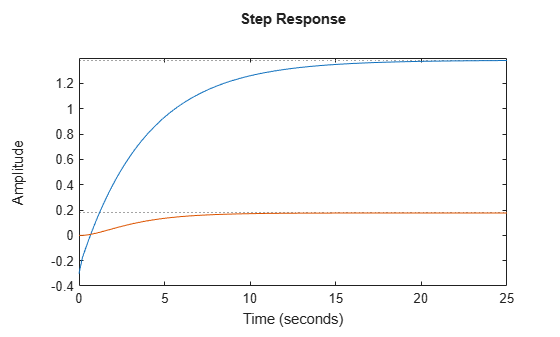Documentation

stepplot

Plot step response and return plot handle

Syntax

h = stepplot(sys)
stepplot(sys,Tfinal)
stepplot(sys,t)
stepplot(sys1,sys2,...,sysN)
stepplot(sys1,sys2,...,sysN,Tfinal)
stepplot(sys1,sys2,...,sysN,t)
stepplot(AX,...)
stepplot(..., plotoptions)
stepplot(..., dataoptions)

Description

h = stepplot(sys) plots the step response of the dynamic system model sys. It also returns the plot handle h. You can use this handle to customize the plot with the getoptions and setoptions commands. Type

help timeoptions

for a list of available plot options.

For multiinput models, independent step commands are applied to each input channel. The time range and number of points are chosen automatically.

stepplot(sys,Tfinal) simulates the step response from t = 0 to the final time t = Tfinal. Express Tfinal in the system time units, specified in the TimeUnit property of sys. For discrete-time systems with unspecified sample time (Ts = -1), stepplot interprets Tfinal as the number of sampling intervals to simulate.

stepplot(sys,t) uses the user-supplied time vector t for simulation. Express t in the system time units, specified in the TimeUnit property of sys. For discrete-time models, t should be of the form Ti:Ts:Tf, where Ts is the sample time. For continuous-time models, t should be of the form Ti:dt:Tf, where dt becomes the sample time of a discrete approximation to the continuous system (see step). The stepplot command always applies the step input at t=0, regardless of Ti.

To plot the step responses of multiple models sys1,sys2,... on a single plot, use:

stepplot(sys1,sys2,...,sysN)

stepplot(sys1,sys2,...,sysN,Tfinal)

stepplot(sys1,sys2,...,sysN,t)

You can also specify a color, line style, and marker for each system, as in

stepplot(sys1,'r',sys2,'y--',sys3,'gx')

stepplot(AX,...) plots into the axes with handle AX.

stepplot(..., plotoptions) customizes the plot appearance using the options set, plotoptions. Use timeoptions to create the options set.

stepplot(..., dataoptions) specifies options such as the step amplitude and input offset using the options set, dataoptions. Use stepDataOptions to create the options set.

Examples

collapse all

Generate a step response plot for two dynamic systems.

h = stepplot(sys1,sys2);Each step response settles at a different steady-state value. Use the plot handle to normalize the plotted response.

setoptions(h,'Normalize','on')Now, the responses settle at the same value expressed in arbitrary units.

Compare the step response of a parametric identified model to a nonparametric (empirical) model, and view their 3-σ confidence regions. (Identified models require System Identification Toolbox™ software.)

Identify a parametric and a nonparametric model from sample data.

sys1 = ssest(z1,4);
sys2 = impulseest(z1);

Plot the step responses of both identified models. Use the plot handle to display the 3-σ confidence regions.

t = -1:0.1:5;
h = stepplot(sys1,sys2,t);
showConfidence(h,3)
legend('parametric','nonparametric')The nonparametric model sys2 shows higher uncertainty.

Load data for estimating a nonlinear Hammerstein-Wiener model.

z = iddata(y,u,0.2,'Name','Two tank system');

z is an iddata object that stores the input-output estimation data.

Estimate a Hammerstein-Wiener Model of order [1 5 3] using the estimation data. Specify the input nonlinearity as piecewise linear and output nonlinearity as one-dimensional polynomial.

sys = nlhw(z,[1 5 3],pwlinear,poly1d);

Create an option set to specify input offset and step amplitude level.

opt = stepDataOptions('InputOffset',2,'StepAmplitude',0.5);

Plot the step response until 60 seconds using the specified options.

stepplot(sys,60,opt);Tips

You can change the properties of your plot, for example the units. For information on the ways to change properties of your plots, see Ways to Customize Plots (Control System Toolbox).